Tweeter Mids Fullrange Woofer no longer available PA Mids Woofer Fullrange no longer available Car Woofer no longer available

## Monacor

Monacor develops and produces products for HiFi, Car HiFi, PA, Multimedia and Home Cinema since 1965. The brands like MONACOR, IMG Stage Line and CARPOWER are well known for their high quality.

## Mids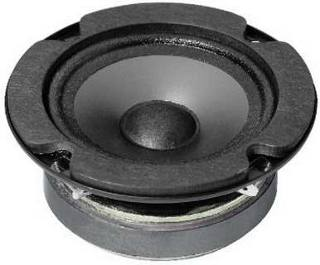open a bigger photo

SPP-90       order no. mo-101150       EUR 18,39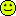excl. VAT: € 15.45 / \$ 17.46
7 cm midrange driver with polypropylene cone.
• power handling (continuous/programme) = 22/45 W
• frequency range = 2000-18000 Hz
• resonance frequency fs = 1000 Hz
• impedance R = 8 Ohm
• sound pressure level SPL = 92 dB (2,83V; 1m)
• DC resistance Re = 6,5 Ohm
• voice coil inductance L = 0,1 mH
• effective piston radiating area Sd = 23 cm2
• voice coil diameter = 15 mm
• mounting diameter d = 61 mm
• overall diameter d = 78 mm
• mounting depth (not countersunk) t = 30 mm
• mass m = 0,3 kg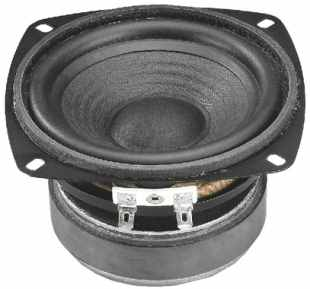SP-100/8       order no. mo-103780       EUR 33,10excl. VAT: € 27.82 / \$ 31.43
10,6 x 10,6 cm midrange driver with paper cone. To avoid compression effects the driver possesses a pole piece hole.
• power handling (continuous/programme) = 30/60 W
• frequency range = 100-7000 Hz
• resonance frequency fs = 61 Hz
• impedance R = 8 Ohm
• sound pressure level SPL = 88 dB (2,83V; 1m)
• DC resistance Re = 6,5 Ohm
• force factor BL = 5,55 N/A
• voice coil inductance L = 0,4 mH
• effective piston radiating area Sd = 52 cm2
• effective mechanical mass incl. air load mms = 5,5 g
• equivalent volume of compliance Vas = 4,7 l
• total Q factor Qts = 0,37 (Qms=2,28, Qes=0,44)
• voice coil diameter = 25 mm
• maximum peak linear excursion vibration xlin = +/- 2,5 mm
• mounting diameter d = 103 mm
• overall diameter d = 106x106 mm
• mounting depth (not countersunk) t = 63 mm
• mass m = 0,8 kgSPH-100C       order no. mo-102300       EUR 68,00excl. VAT: € 57.14 / \$ 64.57
10,6 x 10,6 cm midrange driver with carbon cone. To avoid compression effects the driver possesses a pole piece hole.
• power handling (continuous/programme) = 30/50 W
• frequency range = 60-6000 Hz
• resonance frequency fs = 50 Hz
• impedance R = 8 Ohm
• sound pressure level SPL = 84 dB (2,83V; 1m)
• DC resistance Re = 6 Ohm
• force factor BL = 5,1 N/A
• voice coil inductance L = 0,65 mH
• effective piston radiating area Sd = 54 cm2
• effective mechanical mass incl. air load mms = 7 g
• equivalent volume of compliance Vas = 6 l
• total Q factor Qts = 0,44 (Qms=3,53, Qes=0,5)
• voice coil diameter = 25,5 mm
• maximum peak linear excursion vibration xlin = +/- 4 mm
• mounting diameter d = 96 mm
• overall diameter d = 106x106 mm
• mounting depth (not countersunk) t = 50 mm
• mass m = 0,85 kgrecommended cabinet 1:
closed cabinet with 2,5 L volume
from 116/73 Hz (-3dB/-8dB)

recommended cabinet 2:
9,8 L volume bass reflex cabinet
with HP50 reflex tube, 12 cm long.
from 52/45 Hz (-3dB/-8dB).

SPH-100AL       order no. mo-103810       EUR 54,00excl. VAT: € 45.38 / \$ 51.28
11 cm midrange driver with aluminium cone.
• power handling (continuous/programme) = 40/80 W
• frequency range = 80-5000 Hz
• resonance frequency fs = 80 Hz
• impedance R = 8 Ohm
• sound pressure level SPL = 87 dB (2,83V; 1m)
• DC resistance Re = 6,2 Ohm
• force factor BL = 4,81 N/A
• voice coil inductance L = 0,3 mH
• effective piston radiating area Sd = 55 cm2
• effective mechanical mass incl. air load mms = 4,9 g
• equivalent volume of compliance Vas = 3,2 l
• total Q factor Qts = 0,55 (Qms=3,05, Qes=0,67)
• voice coil diameter = 25 mm
• maximum peak linear excursion vibration xlin = +/- 1,5 mm
• mounting diameter d = 90 mm
• overall diameter d = 124,3 mm
• mounting depth (not countersunk) t = 55 mm
• mass m = 0,65 kgSPH-102KEP       order no. mo-102590       EUR 61,63excl. VAT: € 51.79 / \$ 58.52
10,6 x 10,6 cm midrange driver with kevlar cone. The cone is equipped with a phase plug.
• power handling (continuous/programme) = 40/120 W
• frequency range = 100-10000 Hz
• resonance frequency fs = 95 Hz
• impedance R = 8 Ohm
• sound pressure level SPL = 88 dB (2,83V; 1m)
• DC resistance Re = 7,2 Ohm
• force factor BL = 4,75 N/A
• voice coil inductance L = 0,2 mH
• effective piston radiating area Sd = 55 cm2
• effective mechanical mass incl. air load mms = 3 g
• equivalent volume of compliance Vas = 3 l
• total Q factor Qts = 0,52 (Qms=5,7, Qes=0,57)
• voice coil diameter = 25,5 mm
• maximum peak linear excursion vibration xlin = +/- 1 mm
• mounting diameter d = 95 mm
• overall diameter d = 106x106 mm
• mounting depth (not countersunk) t = 76 mm
• mass m = 0,97 kg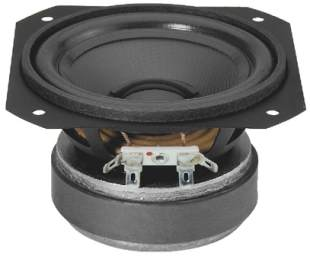SPP-110/4       order no. mo-101050       EUR 34,48excl. VAT: € 28.97 / \$ 32.74
11 x 11 cm midrange driver with synthetics ABS cone. To avoid compression effects the driver possesses a pole piece hole.
• power handling (continuous/programme) = 30/60 W
• frequency range = 70-8000 Hz
• resonance frequency fs = 53 Hz
• impedance R = 4 Ohm
• sound pressure level SPL = 90 dB (2,83V; 1m)
• DC resistance Re = 3,3 Ohm
• force factor BL = 3,3 N/A
• voice coil inductance L = 0,3 mH
• effective piston radiating area Sd = 55 cm2
• effective mechanical mass incl. air load mms = 4,3 g
• equivalent volume of compliance Vas = 8 l
• total Q factor Qts = 0,37 (Qms=2,01, Qes=0,46)
• voice coil diameter = 25,5 mm
• maximum peak linear excursion vibration xlin = +/- 2 mm
• mounting diameter d = 100 mm
• overall diameter d = 110x110 mm
• mounting depth (not countersunk) t = 60 mm
• mass m = 0,9 kg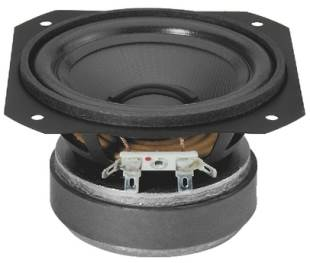SPP-110/8       order no. mo-101060       EUR 34,48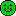excl. VAT: € 28.97 / \$ 32.74
11 x 11 cm midrange driver with synthetics ABS cone. To avoid compression effects the driver possesses a pole piece hole.
• power handling (continuous/programme) = 30/60 W
• frequency range = 70-8000 Hz
• resonance frequency fs = 55 Hz
• impedance R = 8 Ohm
• sound pressure level SPL = 87 dB (2,83V; 1m)
• DC resistance Re = 6,5 Ohm
• force factor BL = 4,6 N/A
• voice coil inductance L = 0,4 mH
• effective piston radiating area Sd = 55 cm2
• effective mechanical mass incl. air load mms = 4,4 g
• equivalent volume of compliance Vas = 8 l
• total Q factor Qts = 0,37 (Qms=2,01, Qes=0,46)
• voice coil diameter = 25,5 mm
• maximum peak linear excursion vibration xlin = +/- 2 mm
• mounting diameter d = 100 mm
• overall diameter d = 110x110 mm
• mounting depth (not countersunk) t = 60 mm
• mass m = 0,9 kgopen a bigger photo

SPH-115       order no. mo-100860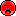- not available
11,5 x 11,5 cm bass-midrange driver with paper cone. The diaphragm is varnished with a hydrophabic coat.
• power handling (continuous/programme) = 50/80 W
• frequency range = 80-8000 Hz
• resonance frequency fs = 65 Hz
• impedance R = 8 Ohm
• sound pressure level SPL = 87 dB (2,83V; 1m)
• DC resistance Re = 5,8 Ohm
• force factor BL = 4,8 N/A
• voice coil inductance L = 0,3 mH
• effective piston radiating area Sd = 55 cm2
• effective mechanical mass incl. air load mms = 4,5 g
• equivalent volume of compliance Vas = 5,5 l
• total Q factor Qts = 0,39 (Qms=2,23, Qes=0,47)
• voice coil diameter = 10 mm
• maximum peak linear excursion vibration xlin = +/- 1,5 mm
• mounting diameter d = 101 mm
• overall diameter d = 115x115 mm
• mounting depth (not countersunk) t = 58 mm
• mass m = 0,55 kgMSH-115       order no. mo-101840       EUR 47,50excl. VAT: € 39.92 / \$ 45.11
11,5 x 11,5 cm midrange driver with high quality cone.
• power handling (continuous/programme) = 50/120 W
• frequency range = 100-10000 Hz
• resonance frequency fs = 85 Hz
• impedance R = 8 Ohm
• sound pressure level SPL = 89 dB (2,83V; 1m)
• DC resistance Re = 6,3 Ohm
• force factor BL = 4,8 N/A
• voice coil inductance L = 0,3 mH
• effective piston radiating area Sd = 55 cm2
• effective mechanical mass incl. air load mms = 4,5 g
• equivalent volume of compliance Vas = 4 l
• total Q factor Qts = 0,43 (Qms=1,98, Qes=0,55)
• voice coil diameter = 25 mm
• maximum peak linear excursion vibration xlin = +/- 1,5 mm
• mounting diameter d = 101 mm
• overall diameter d = 115x115 mm
• mounting depth (not countersunk) t = 55 mm
• mass m = 0,6 kgopen a bigger photo

recommended cabinet 1:
closed cabinet with 1,7 L volume
from 129/81 Hz (-3dB/-8dB)

recommended cabinet 2:
6,8 L volume bass reflex cabinet
with HP50 reflex tube, 15 cm long.
from 58/50 Hz (-3dB/-8dB).

MSH-115HQ       order no. mo-101330       EUR 66,30excl. VAT: € 55.71 / \$ 62.96
10 cm midrange driver with paper cone. The basket is made of die-cast.
• power handling (continuous/programme) = 50/100 W
• frequency range = 100-8000 Hz
• resonance frequency fs = 89 Hz
• impedance R = 8 Ohm
• sound pressure level SPL = 89 dB (2,83V; 1m)
• DC resistance Re = 5,8 Ohm
• force factor BL = 5,35 N/A
• voice coil inductance L = 0,53 mH
• effective piston radiating area Sd = 55 cm2
• effective mechanical mass incl. air load mms = 5,8 g
• equivalent volume of compliance Vas = 2,2 l
• total Q factor Qts = 0,55 (Qms=3,26, Qes=0,66)
• voice coil diameter = 25 mm
• maximum peak linear excursion vibration xlin = +/- 3 mm
• mounting diameter d = 97 mm
• overall diameter d = 116 mm
• mounting depth (not countersunk) t = 64 mm
• mass m = 0,67 kgrecommended cabinet 1:
closed cabinet with 0,79 L volume
from 186/118 Hz (-3dB/-8dB)

recommended cabinet 2:
3 L volume bass reflex cabinet
with HP35 reflex tube, 7 cm long.
from 92/74 Hz (-3dB/-8dB).

MSH-116/4       order no. mo-102150       EUR 49,35excl. VAT: € 41.47 / \$ 46.86
11 cm midrange driver with high quality cone .
• power handling (continuous/programme) = 40/120 W
• frequency range = 120-10000 Hz
• resonance frequency fs = 95 Hz
• impedance R = 4 Ohm
• sound pressure level SPL = 91 dB (2,83V; 1m)
• DC resistance Re = 3,2 Ohm
• force factor BL = 4,3 N/A
• voice coil inductance L = 0,18 mH
• effective piston radiating area Sd = 55 cm2
• effective mechanical mass incl. air load mms = 4,6 g
• equivalent volume of compliance Vas = 2,7 l
• total Q factor Qts = 0,4 (Qms=2,38, Qes=0,48)
• voice coil diameter = 25 mm
• maximum peak linear excursion vibration xlin = +/- 1 mm
• mounting diameter d = 101 mm
• overall diameter d = 124 mm
• mounting depth (not countersunk) t = 55 mm
• mass m = 0,6 kg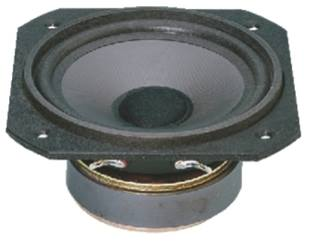MS-125       order no. mo-100250- not available
12,8 x 12,8 cm midrange driver with high quality cone.
• power handling (continuous/programme) = 30/60 W
• frequency range = 1000-7000 Hz
• resonance frequency fs = 550 Hz
• impedance R = 8 Ohm
• sound pressure level SPL = 90 dB (2,83V; 1m)
• mounting diameter d = 108 mm
• overall diameter d = 128x128 mm
• mounting depth (not countersunk) t = 60 mm
• mass m = 0,75 kgopen a bigger photo

SPP-125       order no. mo-101070       EUR 20,43excl. VAT: € 17.17 / \$ 19.40
12 cm midrange driver with polypropylene cone.
• power handling (continuous/programme) = 22/45 W
• frequency range = 800-8000 Hz
• resonance frequency fs = 550 Hz
• impedance R = 8 Ohm
• sound pressure level SPL = 92 dB (2,83V; 1m)
• DC resistance Re = 6,5 Ohm
• voice coil inductance L = 0,2 mH
• effective piston radiating area Sd = 73 cm2
• voice coil diameter = 20 mm
• mounting diameter d = 110 mm
• overall diameter d = 130 mm
• mounting depth (not countersunk) t = 50 mm
• mass m = 0,55 kgMS-130       order no. mo-101400       EUR 44,80excl. VAT: € 37.65 / \$ 42.54
13,5 x 13,5 cm midrange driver with high quality cone. The ribber surround is invers.
• power handling (continuous/programme) = 40/80 W
• frequency range = 200-8000 Hz
• resonance frequency fs = 120 Hz
• impedance R = 8 Ohm
• sound pressure level SPL = 94 dB (2,83V; 1m)
• DC resistance Re = 6,1 Ohm
• force factor BL = 4,2 N/A
• voice coil inductance L = 0,25 mH
• effective piston radiating area Sd = 70 cm2
• effective mechanical mass incl. air load mms = 4 g
• equivalent volume of compliance Vas = 3 l
• total Q factor Qts = 0,88 (Qms=5,23, Qes=1,06)
• voice coil diameter = 25,5 mm
• maximum peak linear excursion vibration xlin = +/- 0,7 mm
• mounting diameter d = 120 mm
• overall diameter d = 135x135 mm
• mounting depth (not countersunk) t = 70 mm
• mass m = 1,3 kg
 Tweeter Mids Fullrange Woofer no longer available PA Mids Woofer Fullrange no longer available Car Woofer no longer available
The cabinet volume calculations are executed considering an external resistance of 0.3 Ohm. We are using, if no other data is known or available, the information supplied by the manufacturer.

Please note: the frequency response doesn't say much about the cut-off frequency inside the cabinet! Therefore, we mostly give you 2 examples with dimensioning considerations and an indication, how low the loudspeaker will reproduce.

Home